# Texas Go Math Grade 4 Lesson 9.2 Answer Key Interpret the Remainder

Refer to our Texas Go Math Grade 4 Answer Key Pdf to score good marks in the exams. Test yourself by practicing the problems from Texas Go Math Grade 4 Lesson 9.2 Answer Key Interpret the Remainder.

## Texas Go Math Grade 4 Lesson 9.2 Answer Key Interpret the Remainder

Essential Question

How can you use remainders in division problems?
Depending on the division problem you are solving, the 4 ways to interpret the remainder are the following.
Write the remainder as a fraction.
Use only the remainder (or drop the quotient) Drop the remainder (or use only the quotient)

Unlock the ProblemMagda has some leftover wallpaper 73 inches long. She wants to cut it into 8 pieces to use arotLnd the photos in her scrapbook. Each piece will have equal length. How long will each piece be?
When you solve a division problem with a remainder, the way you interpret the remainder depends on the situation and the question.
73 ÷ 8
Quotient 9, remainder 1
Explanation:
Magda has some leftover wallpaper 73 inches long.
She wants to cut it into 8 pieces to use arotLnd the photos in her scrapbook.
Each piece will have equal length.
The length of each piece is 9 inches.

One Way Write the remainder as a fraction.

The divisor is _______ pieces.
The __________ is 73 inches.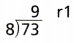Divide to find the quotient and remainder.
The remainder represents 1 inch left over, which can also be divided into 8 equal parts and written as a fraction.
$$\frac{\text { remainder }}{\text { divisor }}$$ = __________
Write the quotient with the remainder written as a fraction. __________
So, each piece will be __________ inches long.
The divisor is 8 pieces.
The dividend is 73 inches.
$$\frac{\text { remainder }}{\text { divisor }}$$ = $$\frac{\text { 1 }}{\text { 8 }}$$
Write the quotient with the remainder written as a fraction. 0.125
So, each piece will be 9.125 inches long.
Explanation:
9 + 0.125 = 9.125
9.125 x 8 = 73

Try This!

Jim made 32 ounces of soup for 3 adults and 2 children. If each person gets the same amount, how many ounces will each person get? Complete the division.Each person gets _________ ounces.
Each person gets 6.4 ounces.
32 ÷ 5
Quotient = 6, remainder = 2
2 ÷ 5 = 0.4
Explanation:
Jim made 32 ounces of soup for 3 adults and 2 children.
If each person gets the same amount,
Each person will get 6.4 or 6 ounces.
32 ÷ 5
Quotient = 6, remainder = 2
2 ÷ 5 = 0.4

Math Talk

Mathematical Processes
Explain what the 2 in the answer represents.
2 is remainder
Explanation:
Jim made 32 ounces of soup for 3 adults and 2 children.
If each person gets the same amount,
Each person will get 6.4 or 6 ounces.
32 ÷ 5
Quotient = 6, remainder = 2
2 ÷ 5 = 0.4

Other Ways

(A) Use only the quotient.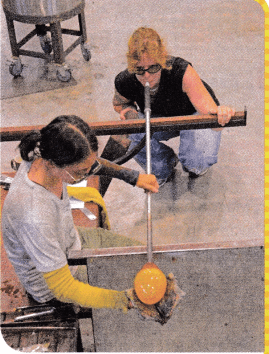Ben is a tour guide at a glass-blowing studio. He can take no ‘more than 7 people at a time on a tour. If 80 people want to see the glass-blowing demonstration, how many groups of 1 people will Ben show around?

First, divide to find the quotient and remainder.
Then, decide how to use the quotient and remainder.
The quotient is _____________ .
The remainder is _____________.Quotient 11
Remainder 3
Explanation:
Ben can give tours to 7 people at a time.
The quotient is the number of tour groups of exactly 7 people he can show around.
80 ÷ 7
11 x 7 = 77
Quotient is 11 and remainder is 3
77 + 3 = 80
The quotient is 11.
The remainder is 3.

So, Ben gives tours to _________ groups of 7 people.
So, Ben gives tours to 11 groups of 7 people.

(B) Add 1 to the quotient.

If Ben gives tours to all 80 people, how many tours will he give? A tour can have no more than 7 people. To show all 80 people around, Ben will have to give 1 more tour.

So, ben will give _________ tours in all for 80 people.
So, ben will give 12 tours in all for 80 people
Explanation:
If Ben gives tours to all 80 people,
A tour can have no more than 7 people.
To show all 80 people around, Ben will have to give 1 more tour.
80 ÷ 7 = 11
3 people left.
Estimate of 12 tours can be done.

(C) Use only the remainder.
Ben gives tours to all 80 people. After he completes the tours for groups of 7 people, how many people are in his last tour? The remainder is 3.
So, Ben’s last tour will have ___________ people.
3 people only
Explanation:
Ben gives tours to all 80 people.
After he completes the tours for groups of 7 people, how many people are in his last tour
The remainder is 3.
80 ÷ 7 = 11
3 people left.

Try This!

Soccer players and their coaches are driven to soccer games in vans. Each van holds 9 people. How many vans are needed for 27 players and 4 coaches?

Divide. ___________ ÷ 9 ___________
Since there are ___________ people left over, ÷ vans are needed to carry the players and coaches.
27 players and 4 coaches = 31 people
31 ÷ 9
The quotient is 3.
The remainder is 4.
Divide. 31 ÷ 9 = 3 is quotient and 4 is remainder
Since there are 4 people left over, 4 vans are needed to carry the players and coaches.
Explanation:
Soccer players and their coaches are driven to soccer games in vans
Each van holds 9 people.
Total vans needed for 27 players and 4 coaches
27 players and 4 coaches = 31 people
31 ÷ 9 = 3 is quotient and 4 is remainder.

Math Talk

Mathematical Processes
Explain why you would not write the remainder as a fraction when you find the number of vans needed.
Each van holds 9 people and 3 vans are needed for 27 players and 1 van for 4 coaches. vans of people can not be divided in to fractions for transport.
Explanation:
A remainder is the number left over after a division.
It has not been divided.
To write a remainder as a fraction, divide it by the number being divided by.

Share and Show

Go Math Lesson 9.2 4th Grade Interpret The Remainder Question 1.
Olivia baked 53 mini-loaves of banana bread to be sliced for snacks at a craft fair. She will place an equal number of loaves in 6 different locations. How many loaves will be at each location?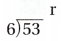8 locations
53 ÷ 6
Quotient is 8 and remainder is 5
Explanation:
Olivia baked 53 mini-loaves of banana bread to be sliced for snacks at a craft fair.
She will place an equal number of loaves in 6 different locations.
Number of loaves in each location
53 ÷ 6
Quotient is 8 and remainder is 5

a. Divide to find the quotient and remainder.
53 ÷ 6
Quotient is 8 and remainder is 5

b. Decide how to use the quotient and remainder to answer the question.
8$$\frac{\text { 5 }}{\text { 6 }}$$

Interpret the remainder to solve.

Question 2.
What if Olivia wants to put only whole loaves at each location? How many loaves. will be at each location?
8 locations

Question 3.
Ed carves 22 small wooden animals to sell at the craft fair. He displays them in rows with 4 animals in a row. How many animals will not be in equal rows?
22 ÷ 4
20 are in 5 rows
2 are not in row
Explanation:
Ed carves 22 small wooden animals to sell at the craft fair.
He displays them in rows with 4 animals in a row.
Number of animals will not be in equal rows
22 ÷ 4
20 are in 5 rows
2 are not in row

Problem Solving

Interpret the remainder to solve.

Question 4.
Myra has a 17-foot roll of crepe paper to make 8 streamers to decorate for a party. How long will each streamer be if she cuts the roll into equal pieces?
2 feet of crepe paper roll
2$$\frac{\text { 1 }}{\text { 8 }}$$
Explanation:
Myra has a 17-foot roll of crepe paper to make 8 streamers to decorate for a party.
How long will each streamer be if she cuts the roll into equal pieces
17 ÷ 8 = 2 is the quotient and 1 is the remainder.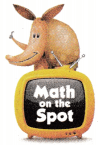H.O.T. Multi-Step Juan has a piano recital next month. Last week he practiced for 8 hours in the morning and 7 hours in the afternoon. A full practice session is 2 hours long. How many hill practice sessions does Juan complete?
7 complete sessions
7$$\frac{\text { 1 }}{\text { 2 }}$$
Explanation:
Last week Juan practiced for 8 hours in the morning and
7 hours in the afternoon.
Total he practiced 8 + 7 = 15 hours.
A full practice session is 2 hours long.
Total practice sessions he completed
15 ÷ 2 = 7 is quotient and 1 is remainder.

Question 6.
H.O.T. A total of 25 students sign up to be hosts on Parents’ Night. Teams of 3 students greet parents. How many students cannot be on a team? How many more students must help so everyone is on a team? Explain.
1 student cannot be on a team.
7 more students must help.
Explanation:
A total of 25 students sign up to be hosts on Parents’ Night.
Teams of 3 students greet parents.
Number of students cannot be on a team is 1
25 ÷ 3 = 8 Quotient
1 remainder
Number of more students must help so everyone is on a team is 7
As, each team contains 8 students
To form one more team we need 7 students.

Question 7.
Apply Jim has a puppet pattern that calls for 6 feet of fleece. He has 50 feet of fleece. If he makes the greatest number of puppets possible, how much fleece will he have left?
(A) 2 feet
(B) 7 feet
(C) 4 feet
(D) 8 feet
Option(A)
Explanation:
Apply Jim has a puppet pattern that calls for 6 feet of fleece.
He has 50 feet of fleece.
Total number of puppets possible,
50 ÷ 6 = 8
The leftover fleece is 2 feet.

Deshawn wants to place 77 photos in an album. Each page of the album holds 9 photos. What is the least number of pages Deshawn will need to use so that all 77 of the photos are placed in the album?
(A) 5 pages
(B) 9 pages
(C) 8 pages
(D) 6 pages
Option(A)
Explanation:
Deshawn wants to place 77 photos in an album.
Each page of the album holds 9 photos.
The least number of pages Deshawn will need to use is 5
77÷ 9 =  72 Quotient
5 is left over pages.

Question 9.
Multi-Step Kathy has 10 tortillas that she will use to make mini pizzas. She has 43 pepperoni slices and will use 7 of them on each pizza. After she makes as many pepperoni pizzas as she can, she will make cheese pizzas. How many cheese pizzas will Kathy make?
(A) 3
(B) 6
(C) 5
(D) 4
Option(D)
Explanation:
Kathy has 10 tortillas that she will use to make mini pizzas.
She has 43 pepperoni slices and will use 7 of them on each pizza.
43÷7 = 6
After she makes as many pepperoni pizzas as she can, she will make cheese pizzas.
Number of cheese pizzas will Kathy make
10 – 6 = 4

TEXAS Test Prep

Mr. Alla gives a “Good Job” badge to each of the 74 students who help at a school event. There are 8 badges in a package. How many packages will he open?
(A) 9$$\frac{1}{4}$$
(B) 9
(C) 2
(D) 10
Option(A)
Explanation:
Mr. Alla gives a “Good Job” badge to each of the 74 students who help at a school event.
There are 8 badges in a package.
Number of packages will he open
74÷8 = 9 Quotient
2 is remainder
So, 9$$\frac{1}{4}$$

### Texas Go Math Grade 4 Lesson 9.2 Homework and Practice Answer Key

Question 1.
The principal at Oak School ordered 25 pizzas for the fourth grade classes to share. He will deliver an equal number of pizzas to each of 8 classes. How many pizzas will each class receive?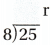a. Divide to find the quotient and remainder.
Quotient =3
Remainder =1

b. Decide how to use the quotient and remainder to answer the question.
25÷8 = 3
Quotient = 3
Remainder = 1
Explanation:
To calculate quotient and remainder:
quotient= dividend / divisor;
remainder= dividend % divisor;

Question 2.
What if the principal wants to deliver only whole pizzas to each class? How many pizzas will each class receive?
Each class will receive 3 pizzas
Explanation:
25÷8 = 3
1 pizza is left over

Question 3.
A total of 58 trophies will be given out at the swim meet. Each box holds 6 trophies, How many boxes are needed to carry the trophies?
Divide 58 ÷ 6 ____________
Since there are ____________ trophies left over, ____________ boxes are needed to carry 58 trophies.
Divide 58 ÷ 6 = 9 is quotient and 6 is remainder
Since there are 6 trophies left over, 9 boxes are needed to carry 58 trophies.
Explanation:
A total of 58 trophies will be given out at the swim meet.
Each box holds 6 trophies,
Total boxes needed to carry the trophies
58÷6 = 9
Left over trophies = 6
4 trophies are less in 10th box.

Problem Solving

Question 4.
Chandra has 15 feet of ribbon to make bows for 6 cheer team members. How much ribbon can she use for each bow if she cuts the ribbon into equal pieces?
2$$\frac{\text { 1 }}{\text { 2 }}$$ feet
Explanation:
Chandra has 15 feet of ribbon to make bows for 6 cheer team members.
Total ribbon she use for each bow if she cuts the ribbon into equal pieces
15÷6 = 2 feet
3feet is left
2$$\frac{\text { 1 }}{\text { 2 }}$$

A group of 34 students will go to the museum. Each van can hold 6 students. What is the least number of vans needed to transport the students?
5 vans are needed
Explanation:
A group of 34 students will go to the museum.
Each van can hold 6 students.
The least number of vans needed to transport the students
34÷6 = 5
4 students are left.

Lesson Check

Question 6.
Hachel wants to place 50 cupcakes in boxes to sell at the school carnival. If each box holds 4 cupcakes, how many boxes can she fill with exactly 4 cupcakes?
(A) 14
(B) 16
(C) 12
(D) 13
Option(C)
Explanation:
Hachel wants to place 50 cupcakes in boxes to sell at the school carnival.
If each box holds 4 cupcakes,
Total boxes she fill with exactly 4 cupcakes
50÷4 = 12
2 cup cakes are left.

Question 7.
At a wedding reception, there are 75 people seated at tables. Each table seats 8 people. How many full tables are there?
(A) 9
(B) 8
(C) 10
(D) 7
Option (D)
Explanation:
At a wedding reception, there are 75 people seated at tables.
Each table seats 8 people.
Number of tables full
75 ÷ 8
Quotient = 9 , remainder 3

Question 8.
Blake guides nature trail walks for groups of no more than 6 people. If 46 people want to go on a walk, how many people will be in a group of less than 6?
(A) 6
(B) 2
(C) 7
(D) 4
Option(C)
Explanation:
Blake guides nature trail walks for groups of no more than 6 people.
If 46 people want to go on a walk,
Number of people will be in a group of less than 6
46÷6
quotient = 7, remainder = 4

Question 9.
Maria has 20 yards of fabric to make 8 tablecloths. How long will each tablecloth be if she cuts the fabric into equal pieces?
(A) 2$$\frac{1}{2}$$ yards
(B) 3 yards
(C) $$\frac{1}{2}$$ yard
(D) 2 yards
Option(A)
Explanation:
Maria has 20 yards of fabric to make 8 tablecloths.
Total tablecloth she cuts the fabric into equal pieces
20÷8
Quotient = 2; Remainder = 4
2$$\frac{1}{2}$$ yards

Multi-Step A total of 56 students signed up to play in a flag football league. If each team has 10 students, how many more students will need to sign up so all of the students can be on a team?
(A) 6
(B) 4
(C) 5
(D) 2
Option(B)
Explanation:
A total of 56 students signed up to play in a flag football league.
If each team has 10 students,
Number of more students need to sign up so all of the students can be on a team
56 ÷10
Quotient = 5 remainder is 6, 4 more required for next

Multi-Step Jerome and Scott are combining their money to buy raffle tickets. Jerome has $7 and Scott has$10. If each ticket costs $3, how many tickets can they buy? (A) 4 (B) 3 (C) 5 (D) 2 Answer: Option (C) Explanation: Jerome has$7 and
Scott has $10. If each ticket costs$3,
$7 +$10 = $17 Total tickets they buy 17÷3 Quotient = 5; Remainder = 2$3 x 5 = \$15